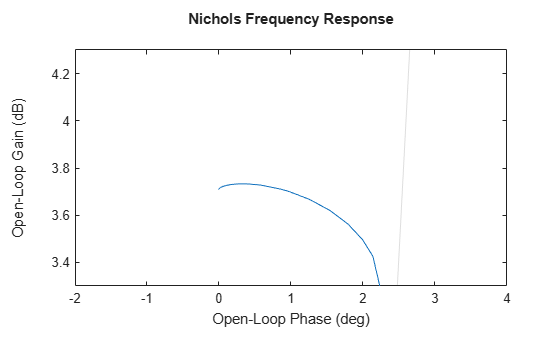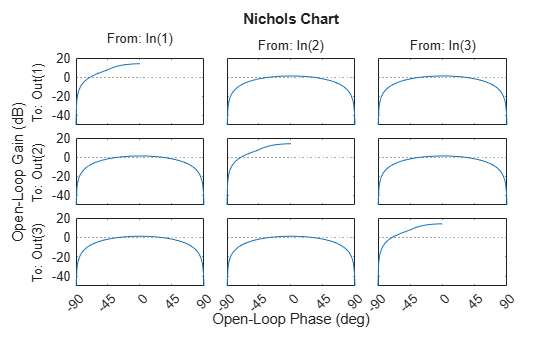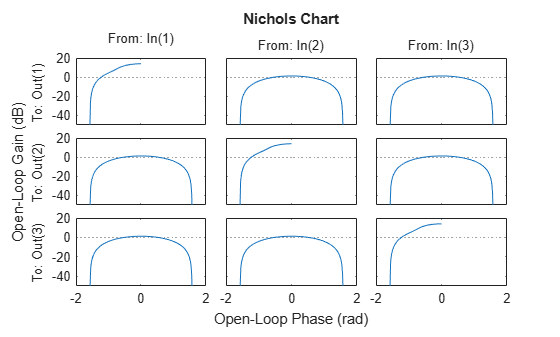# nicholsplot

Plot Nichols frequency responses with additional plot customization options

## Syntax

``h = nicholsplot(sys)``
``h = nicholsplot(sys1,sys2,...,sysN)``
``h = nicholsplot(sys1,LineSpec1,...,sysN,LineSpecN)``
``h = nicholsplot(___,w)``
``h = nicholsplot(AX,___)``
``h = nicholsplot(___,plotoptions)``

## Description

`nicholsplot` lets you plot the Nichols frequency response of a dynamic system model with a broader range of plot customization options than `nichols`. You can use `nicholsplot` to obtain the plot handle and use it to customize the plot, such as modify the axes labels, limits and units. You can also use `nicholsplot` to draw a Nichols plot on an existing set of axes represented by an axes handle. To customize an existing Nichols plot using the plot handle:

1. Obtain the plot handle

2. Use `getoptions` to obtain the option set

3. Update the plot using `setoptions` to modify the required options

For more information, see Customizing Response Plots from the Command Line. To create Nichols plots with default options or to extract the Nichols frequency response data, use `nichols`.

example

````h = nicholsplot(sys)` plots the frequency Nichols response of the dynamic system model `sys` and returns the plot handle `h` to the plot. You can use this handle `h` to customize the plot with the `getoptions` and `setoptions` commands. If `sys` is a multi-input, multi-output (MIMO) model, then `nicholsplot` produces a grid of Nichols plots, each plot displaying the frequency response of one I/O pair.```

example

````h = nicholsplot(sys1,sys2,...,sysN)` plots the Nichols frequency response of multiple dynamic systems `sys1,sys2,…,sysN` on the same plot. All systems must have the same number of inputs and outputs to use this syntax.```

example

````h = nicholsplot(sys1,LineSpec1,...,sysN,LineSpecN)` sets the line style, marker type, and color for the Nichols response of each system. All systems must have the same number of inputs and outputs to use this syntax.```

example

````h = nicholsplot(___,w)` plots Nichols responses for frequencies specified by the frequencies in `w`.If `w` is a cell array of the form `{wmin,wmax}`, then `nicholsplot` plots the response at frequencies ranging between `wmin` and `wmax`.If `w` is a vector of frequencies, then `nicholsplot` plots the response at each specified frequency.You can use `w` with any of the input-argument combinations in previous syntaxes.See `logspace` to generate logarithmically spaced frequency vectors. ```
````h = nicholsplot(AX,___)` plots the Nichols response on the `Axes` object in the current figure with the handle `AX`.```

example

````h = nicholsplot(___,plotoptions)` plots the Nichols frequency response with the options set specified in `plotoptions`. You can use these options to customize the Nichols plot appearance using the command line. Settings you specify in `plotoptions` overrides the preference settings in the MATLAB® session in which you run `nicholsplot`. Therefore, this syntax is useful when you want to write a script to generate multiple plots that look the same regardless of the local preferences.```

## Examples

collapse all

For this example, use the plot handle to change the title, turn on the grid, and set axis limits.

Generate a random state-space model with 5 states and create the Nichols plot with plot handle `h`.

```rng("default") sys = rss(5); h = nicholsplot(sys);```Change the title, enable the grid, and set axis limits. To do so, edit properties of the plot handle, `h` using `setoptions`.

```Title.String = 'Nichols Frequency Response'; setoptions(h,'Title',Title,'Grid','on', 'XLim',{[-2,4]},'YLim',{[3.3,4.3]});```The Nichols plot automatically updates when you call `setoptions`.

Alternatively, you can also use the `nicholsoptions` command to specify the required plot options. First, create an options set based on the toolbox preferences.

`plotoptions = nicholsoptions('cstprefs');`

Change the desired properties of the options set.

```plotoptions.Title.String = 'Nichols Frequency Response'; plotoptions.Grid = 'on'; plotoptions.XLim = {[-2,4]}; plotoptions.YLim = {[3.3,4.3]}; nicholsplot(sys,plotoptions);```You can use the same option set to create multiple Nichols plots with the same customization. Depending on your own toolbox preferences, the plot you obtain might look different from this plot. Only the properties that you set explicitly, in this example `Title`, `Grid`, `XLim` and `YLim`, override the toolbox preferences.

For this example, create a Nichols plot that uses 15-point red text for the title. This plot should look the same, regardless of the preferences of the MATLAB session in which it is generated.

First, create a default options set using `nicholsoptions`.

`plotoptions = nicholsoptions;`

Next, change the required properties of the options set `plotoptions`.

```plotoptions.Title.FontSize = 15; plotoptions.Title.Color = [1 0 0]; plotoptions.FreqUnits = 'Hz'; plotoptions.Grid = 'on';```

Now, create a Nichols plot using the options set `plotoptions`.

`nicholsplot(tf(1,[1,1]),{0,15},plotoptions);`Because `plotoptions` begins with a fixed set of options, the plot result is independent of the toolbox preferences of the MATLAB session.

For this example, create a Nichols plot of the following continuous-time SISO dynamic system. Then, turn the grid on and rename the plot.

`$sys\left(s\right)=\frac{{s}^{2}+0.1s+7.5}{{s}^{4}+0.12{s}^{3}+9{s}^{2}}.$`

Create the transfer function `sys`.

`sys = tf([1 0.1 7.5],[1 0.12 9 0 0]);`

Next, create the options set using `nicholsoptions` and change the required plot properties.

```plotoptions = nicholsoptions; plotoptions.Grid = 'on'; plotoptions.Title.String = 'Nichols Plot of Transfer Function';```

Now, create the Nichols plot with the custom option set `plotoptions`.

`nicholsplot(sys,plotoptions)``nicholsplot` automatically selects the plot range based on the system dynamics.

For this example, consider a MIMO state-space model with 3 inputs, 3 outputs and 3 states. Create a Nichols plot with frequency units in Hz and turn the grid on.

Create the MIMO state-space model `sys_mimo`.

```J = [8 -3 -3; -3 8 -3; -3 -3 8]; F = 0.2*eye(3); A = -J\F; B = inv(J); C = eye(3); D = 0; sys_mimo = ss(A,B,C,D); size(sys_mimo)```
```State-space model with 3 outputs, 3 inputs, and 3 states. ```

Create a Nichols plot with plot handle `h` and use `getoptions` for a list of the options available.

`h = nicholsplot(sys_mimo);``p = getoptions(h)`
```p = FreqUnits: 'rad/s' MagLowerLimMode: 'auto' MagLowerLim: 0 PhaseUnits: 'deg' PhaseWrapping: 'off' PhaseMatching: 'off' PhaseMatchingFreq: 0 PhaseMatchingValue: 0 PhaseWrappingBranch: -180 IOGrouping: 'none' InputLabels: [1x1 struct] OutputLabels: [1x1 struct] InputVisible: {3x1 cell} OutputVisible: {3x1 cell} Title: [1x1 struct] XLabel: [1x1 struct] YLabel: [1x1 struct] TickLabel: [1x1 struct] Grid: 'off' GridColor: [0.1500 0.1500 0.1500] XLim: {3x1 cell} YLim: {3x1 cell} XLimMode: {3x1 cell} YLimMode: {3x1 cell} ```

Use `setoptions` to update the plot with the requires customization.

`setoptions(h,'FreqUnits','Hz','Grid','on');`The Nichols plot automatically updates when you call `setoptions`. For MIMO models, `nicholsplot` produces an array of Nichols plots, each plot displaying the frequency response of one I/O pair.

For this example, compare the Nichols response of a parametric model, identified from input/output data, to a non-parametric model identified using the same data. Identify parametric and non-parametric models based on the data.

Load the data and create the parametric and non-parametric models using `tfest` and `spa`, respectively.

```load iddata2 z2; w = linspace(0,10*pi,128); sys_np = spa(z2,[],w); sys_p = tfest(z2,2);```

`spa` and `tfest` require System Identification Toolbox™ software. The model `sys_np` is a non-parametric identified model while, `sys_p` is a parametric identified model.

Create an options set to turn phase matching and the grid on. Then, create a Nichols plot that includes both systems using this options set.

```plotoptions = nicholsoptions; plotoptions.PhaseMatching = 'on'; plotoptions.Grid = 'on'; plotoptions.XLim = {[-240,0]}; h = nicholsplot(sys_p,'r.-.',sys_np,'b.-.',w,plotoptions); legend('Parametric Model','Non-Parametric model');```## Input Arguments

collapse all

Dynamic system, specified as a SISO or MIMO dynamic system model or array of dynamic system models. Dynamic systems that you can use include:

• Continuous-time or discrete-time numeric LTI models, such as `tf`, `zpk`, or `ss` models.

• Sparse state-space models, such as `sparss` or `mechss` models. Frequency grid `w` must be specified for sparse models.

• Generalized or uncertain LTI models such as `genss` or `uss` (Robust Control Toolbox) models. (Using uncertain models requires Robust Control Toolbox™ software.)

• For tunable control design blocks, the function evaluates the model at its current value to plot the frequency response data.

• For uncertain control design blocks, the function plots the nominal value and random samples of the model.

• Frequency-response data models such as `frd` models. For such models, the function plots the response at frequencies defined in the model.

• Identified LTI models, such as `idtf` (System Identification Toolbox), `idss` (System Identification Toolbox), or `idproc` (System Identification Toolbox) models. For such models, the function can also plot confidence intervals and return standard deviations of the frequency response. (Using identified models requires System Identification Toolbox™ software.)

If `sys` is an array of models, the function plots the frequency responses of all models in the array on the same axes.

Line style, marker, and color, specified as a character vector or string containing symbols. The symbols can appear in any order. You do not need to specify all three characteristics (line style, marker, and color). For example, if you omit the line style and specify the marker, then the plot shows only the marker and no line.

Example: `'--or'` is a red dashed line with circle markers

Line StyleDescription
`-`Solid line
`--`Dashed line
`:`Dotted line
`-.`Dash-dot line
MarkerDescription
`'o'`Circle
`'+'`Plus sign
`'*'`Asterisk
`'.'`Point
`'x'`Cross
`'_'`Horizontal line
`'|'`Vertical line
`'s'`Square
`'d'`Diamond
`'^'`Upward-pointing triangle
`'v'`Downward-pointing triangle
`'>'`Right-pointing triangle
`'<'`Left-pointing triangle
`'p'`Pentagram
`'h'`Hexagram
ColorDescription

`y`

yellow

`m`

magenta

`c`

cyan

`r`

red

`g`

green

`b`

blue

`w`

white

`k`

black

Target axes, specified as an `Axes` object. If you do not specify the axes and if the current axes are Cartesian axes, then `nicholsplot` plots on the current axes. Use `AX` to plot into specific axes.

Nichols plot options set, specified as a `NicholsPlotOptions` object. You can use this option set to customize the Nichols plot appearance. Use `nicholsoptions` to create the option set. Settings you specify in `plotoptions` overrides the preference settings in the MATLAB session in which you run `nicholsplot`. Therefore, `plotoptions` is useful when you want to write a script to generate multiple plots that look the same regardless of the local preferences.

For the list of available options, see `nicholsoptions`.

Frequencies at which to compute and plot frequency response, specified as the cell array `{wmin,wmax}` or as a vector of frequency values.

• If `w` is a cell array of the form `{wmin,wmax}`, then the function computes the response at frequencies ranging between `wmin` and `wmax`.

• If `w` is a vector of frequencies, then the function computes the response at each specified frequency. For example, use `logspace` to generate a row vector with logarithmically spaced frequency values.

Specify frequencies in units of rad/`TimeUnit`, where `TimeUnit` is the `TimeUnit` property of the model.

## Output Arguments

collapse all

Plot handle, returned as a `handle` object. Use the handle `h` to get and set the properties of the Nichols plot using `getoptions` and `setoptions`. For the list of available options, see the Properties and Values Reference section in Customizing Response Plots from the Command Line.

## Version History

Introduced before R2006a